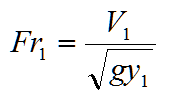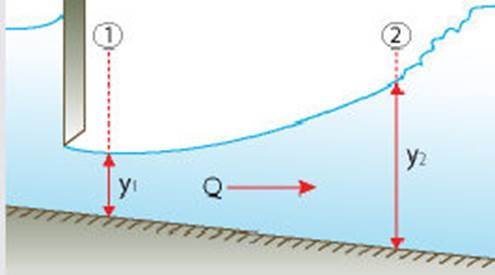#INFOSWMM

# 3.5 Hydraulic Jump

When the flow is Supercritical in an upstream section of a channel and is then forced to become subcritical in a downstream section, a rather abrupt change in depth usually occurs and considerable energy loss accompanies the process. This flow phenomenon, known as hydraulic jump, is described in the following figure.If a hydraulic jump occurs in a rectangular channel, the depth y2 (known as conjugate depth) is expressed as a function of y1 and the Froude number (Fr) as follows:where y2 = water depth in the section 2 ( m, ft)

y1 = water depth in the section 1 (m, ft)

Fr1 = Froude number at the section 1, defined aswhere V1 = upstream velocity [V1 = Q/(y1B)] (m/s, ft/s)

B = channel bottom width (m, ft)

Q = flow (m3/s, ft3/s)

# Hydraulic JumpThe hydraulic jump dialog box is shown below.

## · Input for hydraulic jump:

· Flow Unit – Select the desired flow unit.

· Solving Target – Discharge, upstream depth or downstream depth.

· Upstream Depth – Supercritical flow depth at upstream of the jump.

· Downstream Depth – Subcritical flow depth at downstream of the jump.

· Discharge – Channel flow rate.

· Channel Bottom Width – Bed width of the channel.

## · Output for hydraulic jump:

· Area (Upstream/Downstream) – Flow area at upstream section and downstream section of the jump, respectively.

· Velocity (Upstream/Downstream) – Flow velocity at upstream section and downstream section of the jump, respectively.

· Froude Number (Upstream/Downstream) – Froude number at upstream section and downstream section of the jump, respectively.

· Critical Depth – Flow depth corresponding to the minimum specific energy for the flow rate.

· Head Loss in the Jump – Difference in specific energy at the upstream end and the downstream end of the jump.

Categories: #INFOSWMM, ICM, InfoSWMM